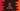# Two different ways to find the circle area in C++# Find the area of a circle in C++ :

We can easily find out the area of a circle in C++. The formula to find the circle area is pi * radius * radius, where pi or π is the mathematical constant and radius is the radius of the circle.

The value π is 3.14159 approximately. In the program, we will take the radius as an input from the user. The user will enter the radius, the program will calculate the area and print it out.

With this program, you will learn how to take user inputs and how to do mathematical calculations in C++.

## C++ program to find the area of a circle :

``````#include <iostream>
using namespace std;

int main()
{
// 1
float PI = 3.14159;
float r, a;

// 2
cout << "Enter the radius : " << endl;
cin >> r;

// 3
a = PI * r * r;

// 4
cout << "Area : " << a << endl;
return 0;
}``````

### Explanation :

The commented numbers in the above program denote the step numbers below :

1. Create one floating-point variable PI to hold the value of π. Create two more float variables r and a to hold the value of radius and area of the circle.
3. Finally, calculate the area using the formula we have discussed above and store that value in a.
4. Print out the result to the user.

### Sample Output :

``````Enter the radius :
6
Area : 113.097

8
Area : 201.062

1
Area : 3.14159``````

If you don’t want to remember the value of PI, you can use the cmath header library. This library comes with a lot of useful mathematical functions and constants. PI is also defined in it. Just import this library using include and use MPI_ in your program.

We can also find the power of a number using the pow method defined in this cmath library. In this program, I will use this method to find the value of radius * radius :

### C++ program using cmath :

``````#include <iostream>
#include <cmath>
using namespace std;

int main()
{
float r, a;

cout << "Enter the radius : " << endl; cin >> r;

a = M_PI * pow(r,2);

cout << "Area : " << a << endl;
return 0;
}``````